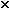# Thermal expansion

• Erenjaeger
In summary, the active element of a laser, made of a 25.0 cm glass rod with a diameter of 1.40 cm, is assumed to have an average coefficient of linear expansion of 9.00 x 10-6 (°C)-1. When the temperature of the rod increases by 64.0°C, the increase in length is 0.0144 cm. Using the equation ΔL = α x L(initial) x ΔT, the increase in diameter can also be calculated. The equation for volume, V = πr^2h, can be used to find the increase in volume by using the new values for length and diameter. It is important to note that the equation for linear expansion can

#### Erenjaeger

A) The active element of a laser is made of a glass rod 25.0 cm long and 1.40 cm in diameter. Assume the average coefficient of linear expansion of the glass is equal to9.0010-6 (°C)−1. The temperature of the rod increases by 64.0°C.
i) What is the increase in length?
I worked this out to be 25.0144cm but doesn't sound right given its original length is 25cm exactly.
ii) What is the increase in its diameter?
iii) What is the increase in its volume?

Can someone pls run me through the working for these three questions.

Hi Erinjaeger. Unfortunately, you will have to show us how you determined your answer. You should also tell us what you think the mathematical relationship is between length, the coefficient of expansion and temperature change.

You should use the homework template to post your question.

AM

Andrew Mason said:
Hi Erinjaeger. Unfortunately, you will have to show us how you determined your answer. You should also tell us what you think the mathematical relationship is between length, the coefficient of expansion and temperature change.

You should use the homework template to post your question.

AM
I used this equation,
ΔL = α x L(initial) x ΔT
Im pretty sure it would be correct and i could work out the iii) part too (i think lol) if i could figure out the ii) part because here v=π r ^2 so V(finial) would use the same equation but different value for the radius right?

Erenjaeger said:
A) The active element of a laser is made of a glass rod 25.0 cm long and 1.40 cm in diameter. Assume the average coefficient of linear expansion of the glass is equal to9.0010-6 (°C)−1. The temperature of the rod increases by 64.0°C.
i) What is the increase in length?
I worked this out to be 25.0144cm but doesn't sound right given its original length is 25cm exactly.
So you're saying it more than doubles in length? I agree that doesn't sound right. (though, your 25.0144cm value is correct for a different question ...)

Erenjaeger said:
I used this equation,
ΔL = α x L(initial) x ΔT
Im pretty sure it would be correct and i could work out the iii) part too (i think lol) if i could figure out the ii) part
That's the right equation, if you want to find the new rod diameter for ii) you just need to use the correct Linitial...

because here v=π r ^2 so V(finial) would use the same equation but different value for the radius right?
π r 2 is an area, not a volume (the units should give it away). (google "volume of a cylinder" if you get stuck)
using A = π d 2 / 4 will get you to your answer for iii) with fewer steps.

whoops i mean the increase worked out to be 0.0144 hence the final length to be 25.0144
and that equation was for the linear expansion ? can i also apply that same equation to the linear expansion of the diameter by using L(initial) as the initial length of the radius?
and for iii) i meant πr^2h which would be right wouldn't it? when using the new value for length or rod ( or height in the equation) and the new radius value ?

Erenjaeger said:
whoops i mean the increase worked out to be 0.0144 hence the final length to be 25.0144
and that equation was for the linear expansion? can i also apply that same equation to the linear expansion of the diameter by using L(initial) as the initial length of the radius?
Yes, the equation holds in all directions. You can pick any two points within the object and find the expansion between those points.

and for iii) i meant πr^2h which would be right wouldn't it?

Yep, but like I hinted previously, there's no reason to use radius at all, you are not given or asked for a radius, using radius just adds unnecessary steps and opportunities for error.

billy_joule said:
Yes, the equation holds in all directions. You can pick any two points within the object and find the expansion between those points.

Yep, but like I hinted previously, there's no reason to use radius at all, you are not given or asked for a radius, using radius just adds unnecessary steps and opportunities for error.
cool thanks a lot man!